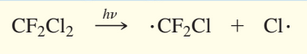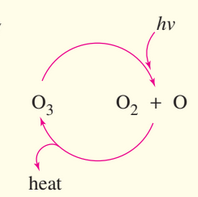×
Get Full Access to Organic Chemistry - 8 Edition - Chapter 4 - Problem 55sp
Get Full Access to Organic Chemistry - 8 Edition - Chapter 4 - Problem 55sp

×

# When healthy, Earth’s stratosphere contains a lowISBN: 9780321768414 33

## Solution for problem 55SP Chapter 4

Organic Chemistry | 8th Edition

• Textbook Solutions
• 2901 Step-by-step solutions solved by professors and subject experts
• Get 24/7 help from StudySoup virtual teaching assistantsOrganic Chemistry | 8th Edition

4 5 1 289 Reviews
13
0
Problem 55SP

When healthy, Earth’s stratosphere contains a low concentration of ozone $$\left(O_{3}\right)$$ that absorbs potentially harmful ultraviolet $$(U V)$$ radiation by the cycle shown at right.

Chlorofluorocarbon refrigerants, such as Freon 12 $$\left(C F_{2} C l_{2}\right)$$, are stable in the lower atmosphere, but in the stratosphere they absorb high-energy UV radiation to generate chlorine radicals.The presence of a small number of chlorine radicals appears to lower ozone concentrations dramatically. The following reactions are all known to be exothermic (except the one requiring light) and to have high rate constants. Propose two mechanisms to explain how a small number of chlorine radicals can destroy large numbers of ozone molecules. Which of the two mechanisms is more likely when the concentration of chlorine atoms is very small?

Equation Transcription:Text Transcription:

(O3)

(UV)

(CF2Cl2)

Step-by-Step Solution:

Solution:

Step 1

All the given reactions are exothermic (except the one requiring light) and to have high rate constants.Here we have to propose two mechanisms to explain how a small number of chlorine radicals can destroy large numbers of ozone molecules.

Mechanism:-1

The 1st mechanism involves the reaction between chlorine free radical with ozone. The reaction has been carried out by three steps as given below:-Among all the three steps, in the 2nd step the concentration of Cl atom is very small, hence the concentration of ClO will also decrease. Although the given mechanism shows catalytic cycle with Cl. but the probability of finding two ClO radicals is zero.

Step 2 of 2

##### Edition: 8
The full step-by-step solution to problem: 55SP from chapter: 4 was answered by , our top Chemistry solution expert on 05/06/17, 06:41PM. This full solution covers the following key subjects: chlorine, Radicals, Small, ozone, radiation. This expansive textbook survival guide covers 25 chapters, and 1336 solutions. Organic Chemistry was written by and is associated to the ISBN: 9780321768414. The answer to “?When healthy, Earth’s stratosphere contains a low concentration of ozone $$\left(O_{3}\right)$$ that absorbs potentially harmful ultraviolet $$(U V)$$ radiation by the cycle shown at right.Chlorofluorocarbon refrigerants, such as Freon 12 $$\left(C F_{2} C l_{2}\right)$$, are stable in the lower atmosphere, but in the stratosphere they absorb high-energy UV radiation to generate chlorine radicals. The presence of a small number of chlorine radicals appears to lower ozone concentrations dramatically. The following reactions are all known to be exothermic (except the one requiring light) and to have high rate constants. Propose two mechanisms to explain how a small number of chlorine radicals can destroy large numbers of ozone molecules. Which of the two mechanisms is more likely when the concentration of chlorine atoms is very small?Equation Transcription: Text Transcription:(O3) (UV) (CF2Cl2)” is broken down into a number of easy to follow steps, and 129 words. This textbook survival guide was created for the textbook: Organic Chemistry, edition: 8. Since the solution to 55SP from 4 chapter was answered, more than 452 students have viewed the full step-by-step answer.